# LOG#220. Higgs&Symmetries.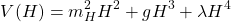Why the leaves are green? Why the sky is blue? Why diamonds are hard? The Quantum Mechanics can indeed answer those questions, and even more subtle questions. The Standard Model (SM) is the frontier in our knowledge of microscopic things, that is, the SM represents the superior quantum theory explaining the building blocks of the Universe (well, a 5% of it actually, but it does not matter for the purposes of this new blog post).

• Six leptons (plus their antiparticles):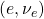,,.
• Six quarks (plus their antiparticles times 8, color factor):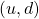,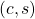,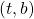.
• Four bosons (up to gauge charges and their antiparticles):.
• The mass giver, the Higgs field,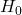, for elementary particles. Gluons and photons are higgs-transparent, bosons that interact more strongly with the Higgs field are more massive.

Timeline(short review):

• 1932: there are protons, electrons, the neutron and the positron.
• 1937: the muon is discovered.
• 1940s: hadron explosion. Pions, kaons, lambdas, deltas, sigmas and other exotic states are discoverd. Puzzlement.
• 1970s: quark theory (aces). Previous S-matrix approaches are substituted by the QCD gauge theory.
• 1980s: gauge bosons discovered.
• 1987: supernova in Magallanic Clouds. Neutrinos come first than photons at SuperKamiokande.
• 1995: top quark is found.
• 1998: neutrino oscillations are confirmed. Neutrinos are massive. First evidence that there is something beyond the SM.  Dark energy is found.
• 2012: Higgs-like boson discovered at the LHC. No new physics signal is found, circa 2019.

Interactions and forces keep united together everything. Why there are 3 generations with increasing mass? Flavor problem. Why the Higgs mass or the electroweak scale so small compared with the Planck mass/scale? Hierarchy problem. Little hierarchy problem: why are neutrinos lighter than other SM particles? Why relative forces between interactions are the way we measure them? Pions are indeed like Van der Waals forces like the one for atomic nuclei. Pion theory is an effective theory for nuclear forces.

There are 4 forces with messengers or messenger particles: gluons, photons, W and Z bosons, Higgs particles and the graviton. Should we consider the mass giver Higgs like another interaction? Particles are classified by angular momentum: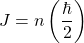for any entire number. Then, there are entire half-spin particles (following the Pauli exclusion principle), and there are entire-spin particles. These are fermions and bosons, matter particles and force messengers. How did interactions arise from fields? The keyword is symmetry. I am not going to talk about SUSY and why it is inevitable in some form we do not know today, but I will tell you about symmetry and its consequences: conservation laws. Indeed, Emmy Noether two theorems go even beyond simple conservation laws. E. Noether two theorems are about invariance under finite and infinite symmetry groups. Global symmetries give you conserved quantities, local (infinite dimensional) symmetries give you identities between field equations/equations of motion for particles/fields. Likely, Noether’s theorems are the 2 most beautiful theorems in mathematical physics (if you know any other theorem rivaling them, let me know!).

Have you ever wonder why energy is conserved? Let me give you some hints:

Example 1. Free particles.

Energy is conserved. There are symmetries under translations in time! Suppose there is a particle with mass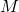. Take the Newton law:Thus,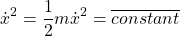Name that constant kinetic energy, or total energy, so you get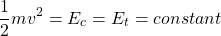Simple and beautiful. Energy is something that is conserved when you have something that is invariant under temporal translations, i.e., motion in time.

Example 2. Motion under constant force.

Generalize the above example to the motion of a free particle under constant force. Then, you get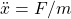Then, again this is invariant under shifts in time, and there is a conserved energy function, namely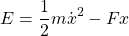Check:Now, use the equation of motion above, and you effectively get that. Energy is conserved again! This is important in the case of gravitational field near the surface and other examples!

Example 3. Motion of simple harmonic oscillators.

Now, you get the equation of motion (EOM):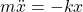Again, under time translations, the EOM is invariant, so there is some energy functional. It yields the classical formula holds: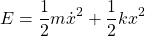Check:Example 4. Motion in a gravitational (newtonian) field.Again, the classical energy function (by time invariance) reads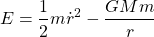Check of constancy, using the EOM as above: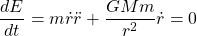Symmetries or invariance are tied deeper into conservation laws by the Noether first theorem:

• Temporal translation invariance implies energy conservation.
• Invariance under spatial translations imply linear momentum conservation as well.
• Rotational invariance means conservation of angular momentum, certain bivector or antisymmetric matrix in higher dimensions.
• Changes in reference frames are tied to conservation of invariant mass and spin of particles. Boosts or Lorentz transformations have certainly non-trivial conservation laws (a center of mass-like conservation law). Boosts in galilean relativity has motion of center of mass constancy under these transformations.
• Higher non-trivial symmetries have correspondingly conservation laws. Some non trivial examples are the Kepler problem, string theory or general relativity theories. Also, invariance under scale transformations have conservation laws.

Remark: under boosts (SR), you get the conserved quantities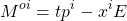Remark(II): discrete symmetries, produce multiplicative conservation laws of parity (P), charge conjugation (C) and time reversal (T). Particles are usually classified in the Particle Data Booklet by.

Remark(III): hidden anomalous symmetries in the SM are baryon number or lepton number conservation. Beyond the Standard Model, these numbers can be violated and thus, proton or other generally stable particles could decay. Experimentally, fortunately for us,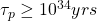. The proton is the lightest particle with baryon number not zero, so it can not decay. Baryon number is usually defined as. Muons are unstable in microseconds, rho particles are unstable in yoctoseconds.

What happens with internal (non-space-time) symmetries? In any quantum theory and Quantum Field Theory, global internal symmetries are associated to conservation laws and quantum numbers. Even discrete symmetries have conserved quantities (discrete and multiplicative quantities inded). Particles are field excitations. So, how internal symmetry arise in field equations. Let me assumeand. Suppose a symmetry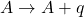and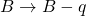is certain symmetry. The field equation is: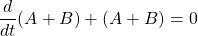Under global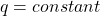symmetry transformations, you can check easily the invariance of the above field equation. Local field symmetries imply the existence of compensating fields and identities called Noether identities between field equations. That local gauge invariance implies the existence of gauge fields is a tryumph of modern mathematical physics, and it is due to Noether in the end. Under LOCAL gauge transformations,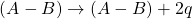And thus, the above EOM is NOT invariant. However, you can RESTORE invariance introducing gauge (compensating) fields. How????? Let me show you a Dirac-like equation example. Write the EOMThe local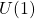invariance under phase transformations of the wave function or field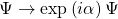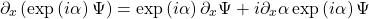You can check that the Dirac equation above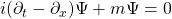is NOT locally gauge invariant. You must introduce a new field, the gauge field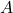, transforming under symmetry as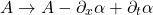Then, a modified field theory (charged) arise, that IS invariant under gauge transformations. Let us do the calculations explictly: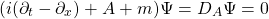under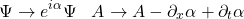transforms and you get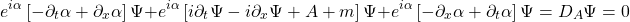Local invariance holds now!!!!!!!This U(1) trick is mimicked for SU(2) and SU(3) symmetries (non-abelian) and weak charge (flavor) and color charge are thus related to non-abelian gauge invariances! A problem arises, however, within the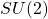case (not in the color force case). The symmetries of the W and Z, when local, have a bad behaviour when mass is present. In other words, the mass of the gauge bosons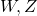spoils gauge invariance. That is where Higgs fields and the Higgs mechanics arise. In 1964, Higgs (and indepently other researches, by the particles are due to Higgs himself, with Nobel Prize merits) introduced a new field and new particles to restore weak gauge invariance when mass terms are present. The name spontaneous symmetry breaking or hidden symmetry is also used here. The ideas are powerful:

• There is a new field, the Higgs field, permeating the space-time, like a fluid. Mass is similar to friction with this relativistic field.
• Associated to the Higgs field, there are excitations of the field, called Higgs particles. Higgs particles are waves in the Higgs field, and these waves give masses to interacting particles with the Higgs. Transparent particles to the Higgs fields get no masses.
• To get a Higgs field requires a lot of concentrated energy.

Simplest Higgs set-up:

Take an electron field,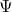(it can also be a boson field like the W or Z).

Under gauge symmetry, it transforms as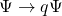and its mass term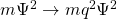. Here,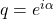. Thus, mass term is not invariant under gauge local transformations. Define H such as under symmetry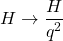Note that we will get gauge invariance plus an interaction of type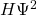. Then, the productis invariant under gauge transformations. Expand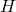around a vacuum as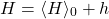then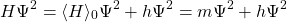where we can define “mass” as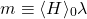and the Higgs coupling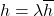In general,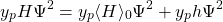Thus, you get a generic mass term for ANY fundamental (elementary, not composite) particle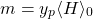In other words,Experimentally, the Higgs v.e.v is about 246 GeV, and it was know much before the Higgs boson discovery in 2012. However, some issues in the SM can not be solved:

• The Higgs field introduces introduces new Yukawa interactions not coming from any SM symmetry.
• The Higgs mass itself is not fixed by any SM symmetry. Indeed, it could have been in principle very heavy, but some theoretical arguments were known before the Higgs discovery. It the Higgs existed, it could not be very heavy without spoiling the properties of the known SM. You should have invented other mechanism if the Higgs field were not been found at the LHC. SSB and Higgs fields could not be too heavy. However, something protects the Higgs to become heavy from loop corrections to mass. What it is? We do know. It could be supersymmetry (SUSY) or any other new kind of symmetry.
• Higgs field is close to be metastable in about. New particles can stabilize the Higgs vacuum, but it is a hard problem!
• The Higgs field can NOT be the dark energy, it is too heavy. However, the Higgs field could be the inflaton. No proof of this is known, it is speculative.
• The Higgs field is not consistent with the known value of the cosmological constant. However, we can not be eager here, since we do not know what dark energy is and we do not what the Higgs-like partice truly is. GR is not a quantum theory, not a YM theory at least, and we do not know how to make GR consistent with the SM. That is why they are usually considered apart to each other.
• The searches for any theory beyond the SM, BSM theories, like string theory or loop quantum gravity, is being guided by the same principles that Maxwell used to derive his electromagnetic synthesis in the 19th century. Similarly, Einstein was guided by symmetry to SR and to GR from simple principles. The SM and the GR are believed to be effective field theories, approximate theories at low energies. We need GUTs or TOE for a further final unification.
• The origin of mass is now turned into a problem of why Yukawa Higgs couplings are the values we observed. Even the Higgs field has its own self-coupling. We need a better theory to understand the origin of mass.

What is the future of fundamental physics? Expensive experiments and cheap experiments. Brilliant minds observing the Universe with new tools. Philosophy is not useful as before. Scientific advances require feedback from theory and experiment. New colliders (CLIC, the muon collider, the Chinese 100TeV collider, the FCC,…) will require complementary projects and dark matter/dark energy extra experiments. Gravitational waves, gamma ray astronomy, neutrino astronomy and multimessenger astronomy is a new exciting field. Theoretical speculations, like those guided by philosophy, are not useful without experimental support. We need data and test hypotheses!

This site uses Akismet to reduce spam. Learn how your comment data is processed.